# ML Aggarwal Solutions for Class 9 Maths Chapter 18 Trigonometric Ratios and Standard Angles

ML Aggarwal Solutions for Class 9 Maths Chapter 18 Trigonometric Ratios and Standard Angles provide detailed solutions to the chapter test problems. The solutions are created by individual subject experts at BYJU’S following the latest ICSE guidelines. In order to secure high marks in Mathematics, practising to solve problems on a daily basis is important. Students can access the solutions of the chapter test question from the ML Aggarwal Solutions for Class 9 Maths Chapter 18 Trigonometric Ratios and Standard Angles PDF, which is available in the link attached below.

Chapter 18 contains problems with regard to proving trigonometric identities and solving trigonometric equations using the trigonometric ratios of standard angles. ML Aggarwal solutions are readily available in PDF format so that the students can make use of them offline while solving the exercise problems of ML Aggarwal textbook. With the help of this resource, students will be able to improve their problem-solving skills and also learn to manage time by learning simple tricks and shortcuts embedded in these solutions.

## ML Aggarwal Solutions for Class 9 Maths Chapter 18 Trigonometric Ratios and Standard Angles Download PDF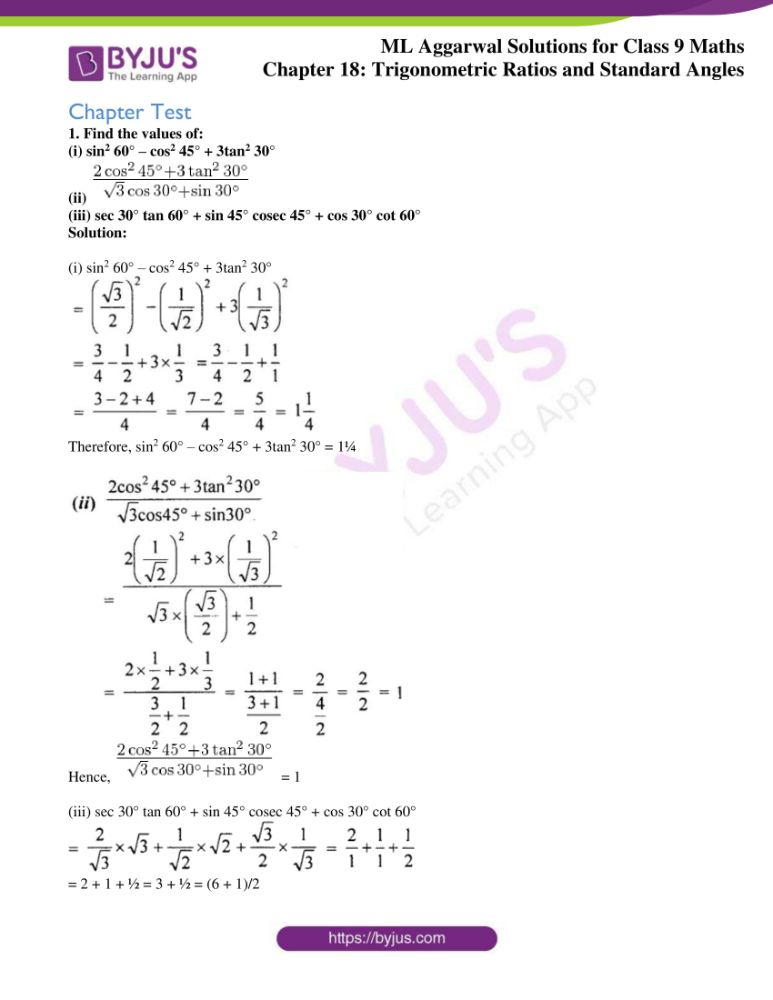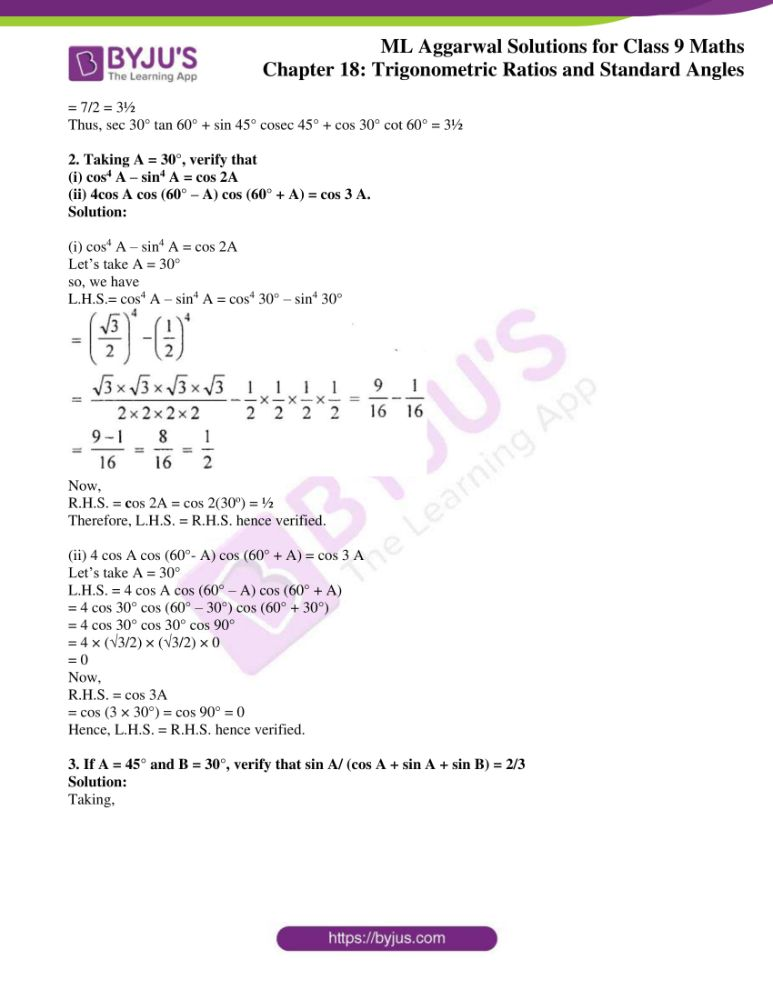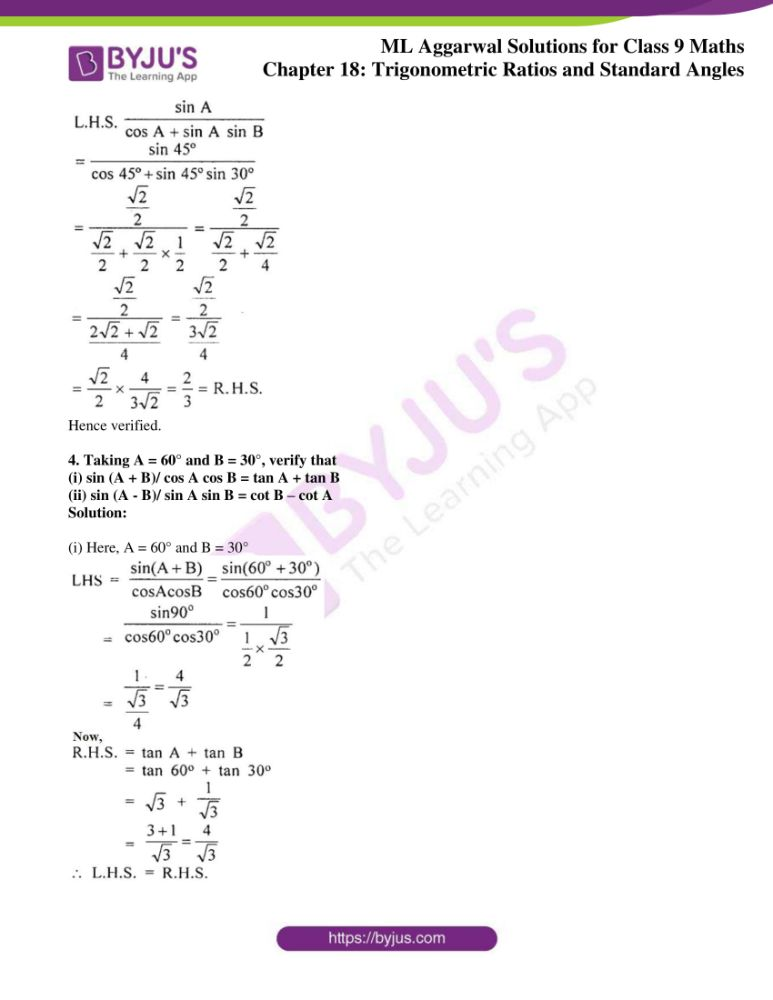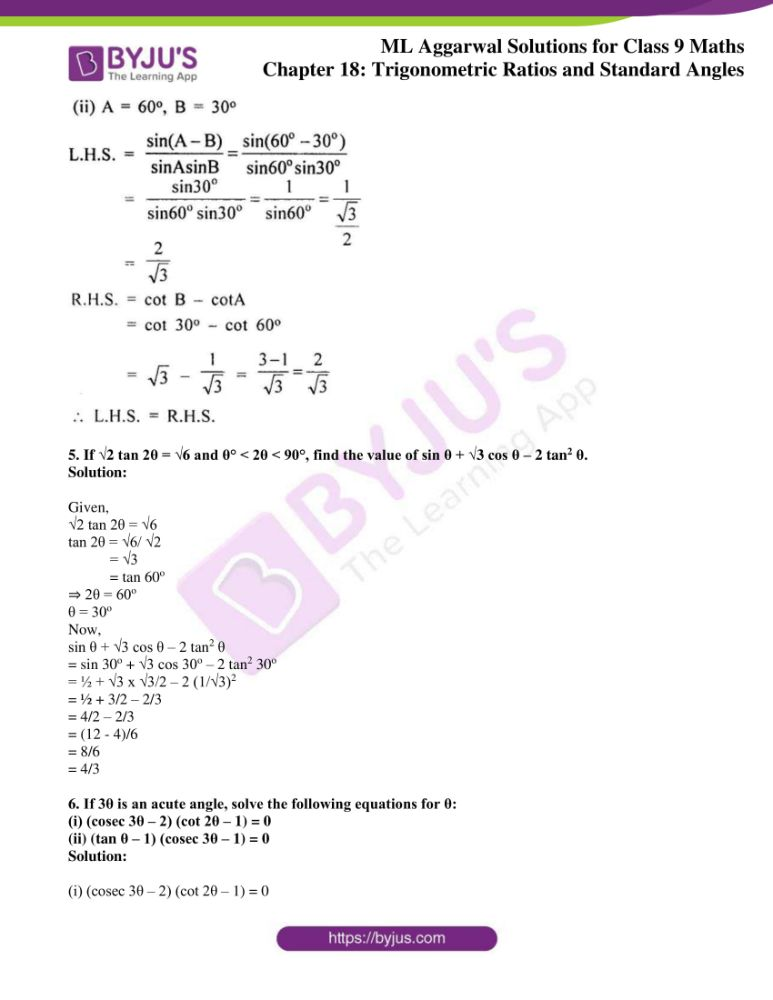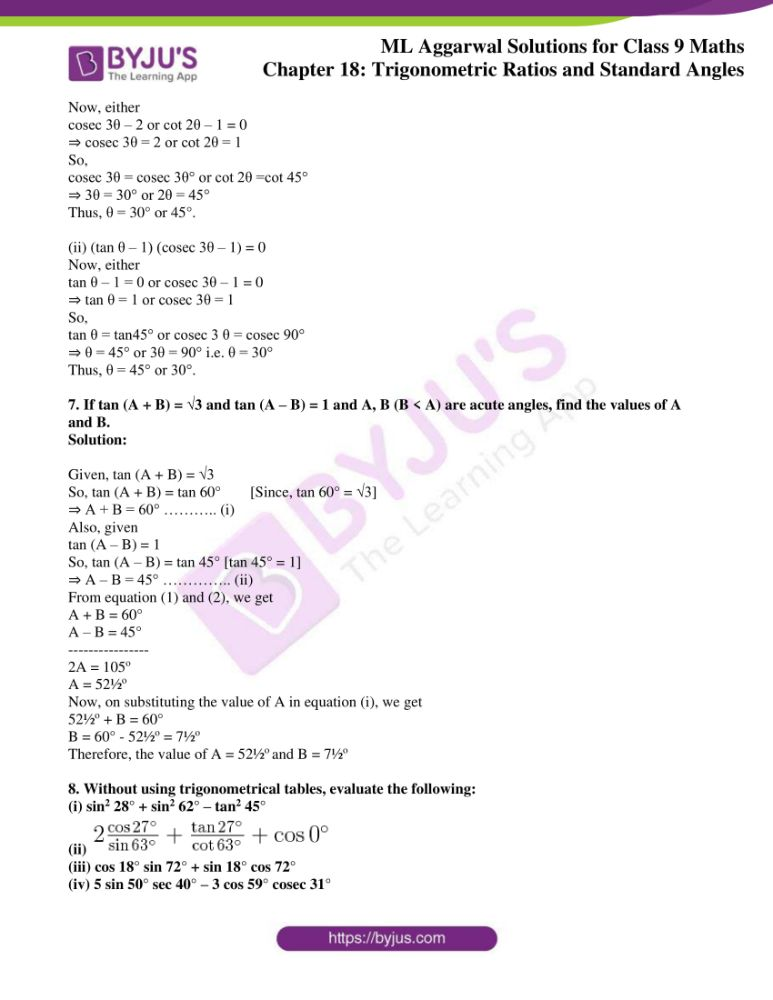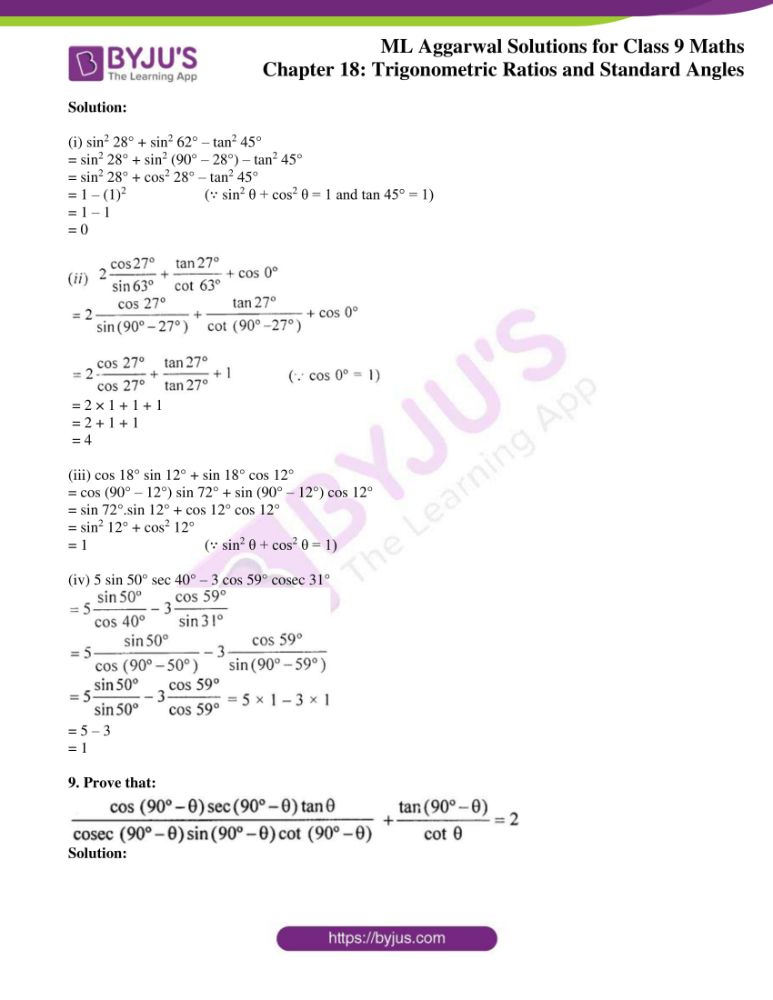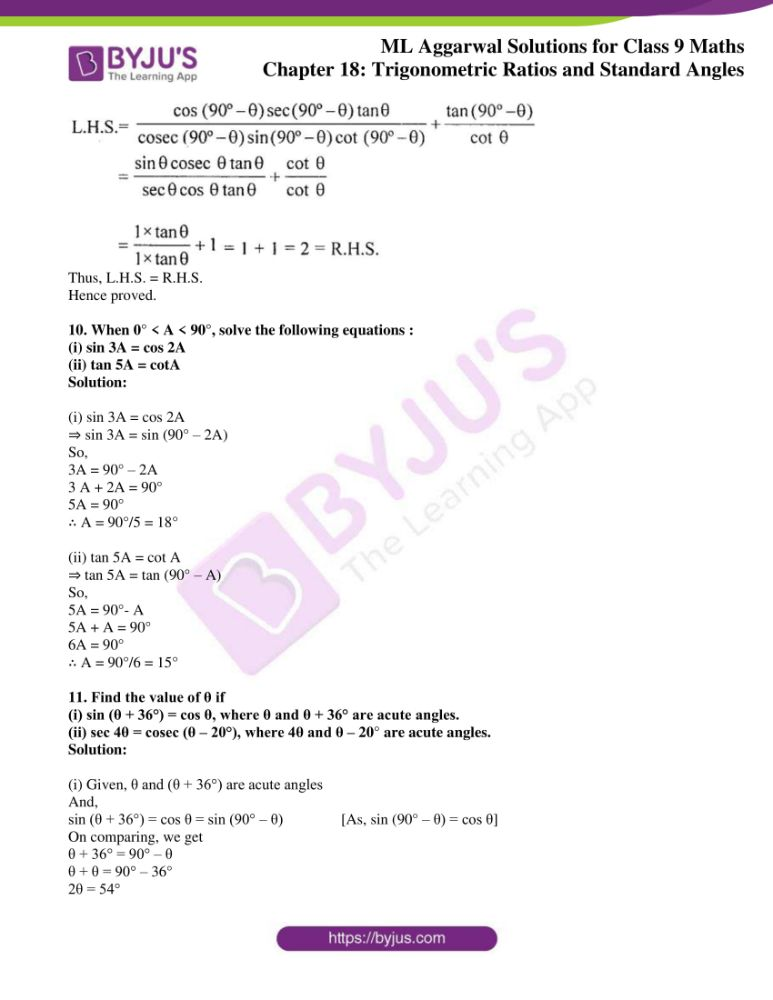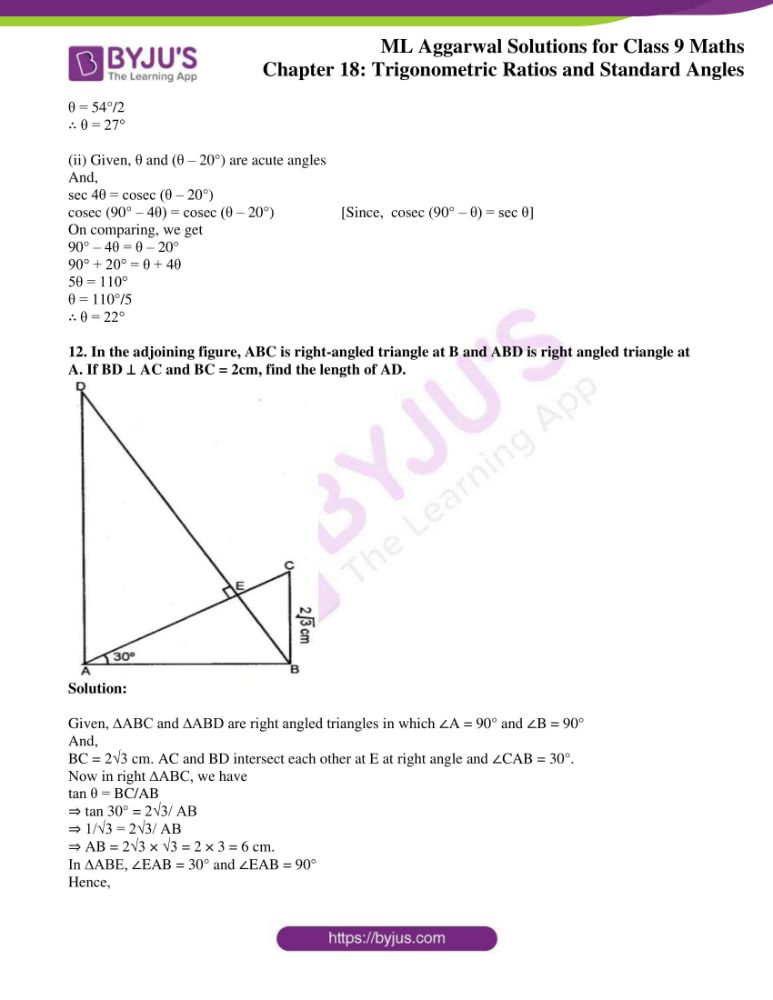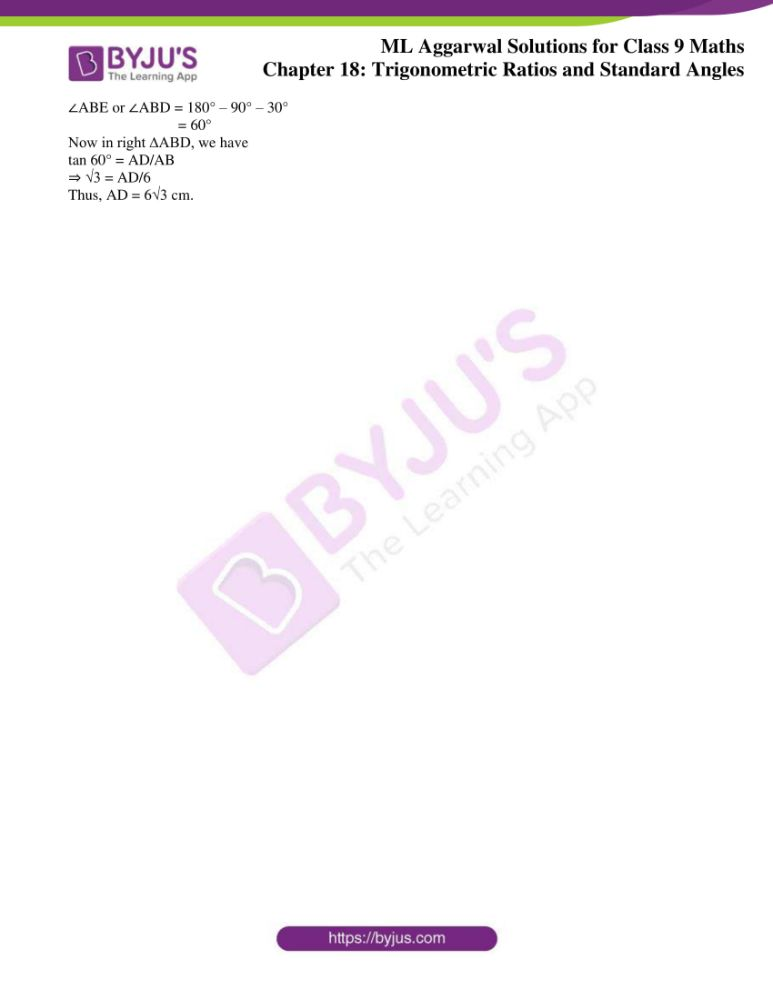## Access ML Aggarwal Solutions for Class 9 Maths Chapter 18 Trigonometric Ratios and Standard Angles

Chapter Test

1. Find the values of:
(i) sin2 60° – cos2 45° + 3tan2 30°
(ii)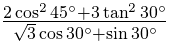(iii) sec 30° tan 60° + sin 45° cosec 45° + cos 30° cot 60°

Solution:

(i) sin2 60° – cos2 45° + 3tan2 30°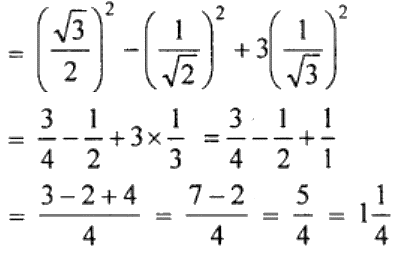Therefore, sin2 60° – cos2 45° + 3tan2 30° = 1¼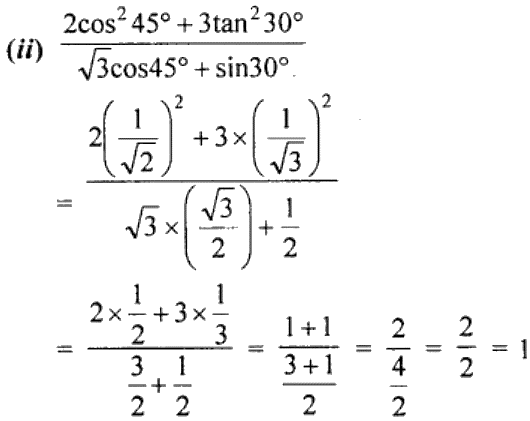Hence,= 1

(iii) sec 30° tan 60° + sin 45° cosec 45° + cos 30° cot 60°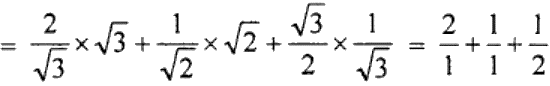= 2 + 1 + ½ = 3 + ½ = (6 + 1)/2

= 7/2 = 3½

Thus, sec 30° tan 60° + sin 45° cosec 45° + cos 30° cot 60° = 3½

2. Taking A = 30°, verify that
(i) cos4 A – sin4 A = cos 2A
(ii) 4cos A cos (60° – A) cos (60° + A) = cos 3 A.

Solution:

(i) cos4 A – sin4 A = cos 2A

Let’s take A = 30°

so, we have

L.H.S.= cos4 A – sin4 A = cos4 30° – sin4 30°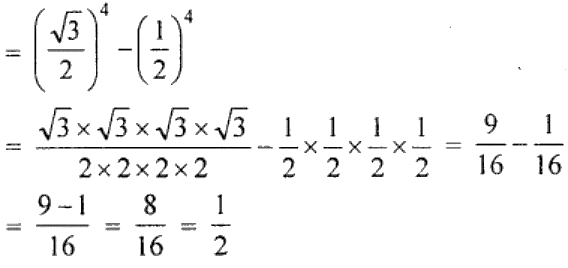Now,

R.H.S. = cos 2A = cos 2(30o) = ½

Therefore, L.H.S. = R.H.S. hence verified.

(ii) 4 cos A cos (60°- A) cos (60° + A) = cos 3 A

Let’s take A = 30°

L.H.S. = 4 cos A cos (60° – A) cos (60° + A)

= 4 cos 30° cos (60° – 30°) cos (60° + 30°)

= 4 cos 30° cos 30° cos 90°

= 4 × (√3/2) × (√3/2) × 0

= 0

Now,

R.H.S. = cos 3A

= cos (3 × 30°) = cos 90° = 0

Hence, L.H.S. = R.H.S. hence verified.

3. If A = 45° and B = 30°, verify that sin A/ (cos A + sin A + sin B) = 2/3

Solution:

Taking,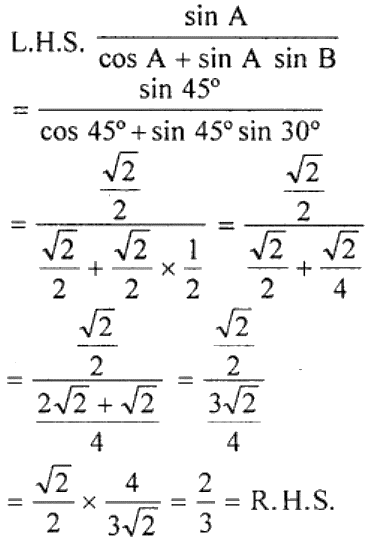Hence verified.

4. Taking A = 60° and B = 30°, verify that

(i) sin (A + B)/ cos A cos B = tan A + tan B

(ii) sin (A – B)/ sin A sin B = cot B – cot A

Solution:

(i) Here, A = 60° and B = 30°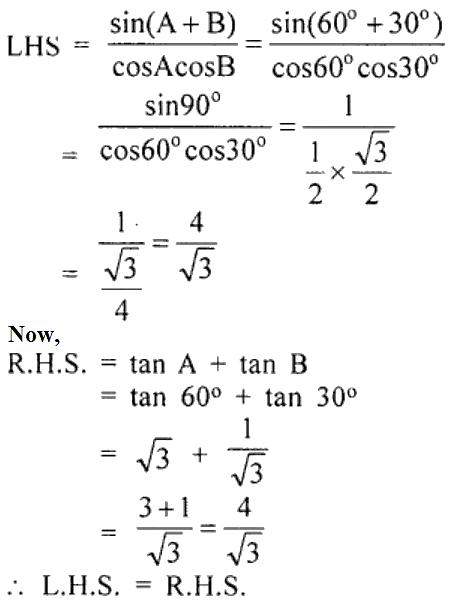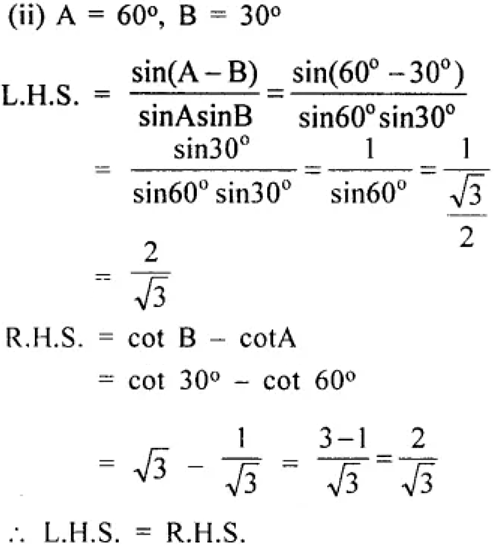5. If √2 tan 2θ = √6 and θ° < 2θ < 90°, find the value of sin θ + √3 cos θ – 2 tan2 θ.

Solution:

Given,

√2 tan 2θ = √6

tan 2θ = √6/ √2

= √3

= tan 60o

⇒ 2θ = 60o

θ = 30o

Now,

sin θ + √3 cos θ – 2 tan2 θ

= sin 30o + √3 cos 30o – 2 tan2 30o

= ½ + √3 x √3/2 – 2 (1/√3)2

= ½ + 3/2 – 2/3

= 4/2 – 2/3

= (12 – 4)/6

= 8/6

= 4/3

6. If 3θ is an acute angle, solve the following equations for θ:
(i) (cosec 3θ – 2) (cot 2θ – 1) = 0

(ii) (tan θ – 1) (cosec 3θ – 1) = 0

Solution:

(i) (cosec 3θ – 2) (cot 2θ – 1) = 0

Now, either

cosec 3θ – 2 or cot 2θ – 1 = 0

⇒ cosec 3θ = 2 or cot 2θ = 1

So,

cosec 3θ = cosec 3θ° or cot 2θ =cot 45°

⇒ 3θ = 30° or 2θ = 45°

Thus, θ = 30° or 45°.

(ii) (tan θ – 1) (cosec 3θ – 1) = 0

Now, either

tan θ – 1 = 0 or cosec 3θ – 1 = 0

⇒ tan θ = 1 or cosec 3θ = 1

So,

tan θ = tan45° or cosec 3 θ = cosec 90°

⇒ θ = 45° or 3θ = 90° i.e. θ = 30°

Thus, θ = 45° or 30°.

7. If tan (A + B) = √3 and tan (A – B) = 1 and A, B (B < A) are acute angles, find the values of A and B.

Solution:

Given, tan (A + B) = √3

So, tan (A + B) = tan 60° [Since, tan 60° = √3]
⇒ A + B = 60° ……….. (i)

Also, given

tan (A – B) = 1

So, tan (A – B) = tan 45° [tan 45° = 1]
⇒ A – B = 45° ………….. (ii)

From equation (1) and (2), we get

A + B = 60°

A – B = 45°

—————-

2A = 105o

A = 52½o

Now, on substituting the value of A in equation (i), we get

52½o + B = 60°

B = 60° – 52½o = 7½o

Therefore, the value of A = 52½o and B = 7½o

8. Without using trigonometrical tables, evaluate the following:
(i) sin2 28° + sin2 62° – tan2 45°
(ii)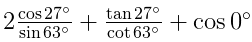(iii) cos 18° sin 72° + sin 18° cos 72°
(iv) 5 sin 50° sec 40° – 3 cos 59° cosec 31°

Solution:

(i) sin2 28° + sin2 62° – tan2 45°

= sin2 28° + sin2 (90° – 28°) – tan2 45°

= sin2 28° + cos2 28° – tan2 45°

= 1 – (1)2  (∵ sin2 θ + cos2 θ = 1 and tan 45° = 1)

= 1 – 1

= 0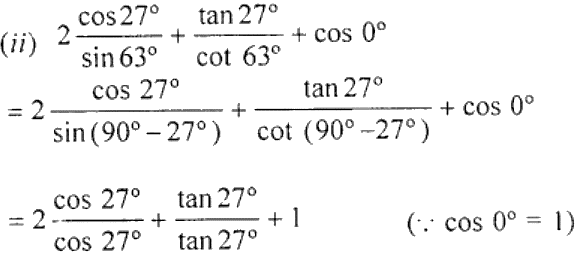= 2 × 1 + 1 + 1

= 2 + 1 + 1

= 4

(iii) cos 18° sin 12° + sin 18° cos 12°

= cos (90° – 12°) sin 72° + sin (90° – 12°) cos 12°

= sin 72°.sin 12° + cos 12° cos 12°

= sin2 12° + cos2 12°

= 1 (∵ sin2 θ + cos2 θ = 1)

(iv) 5 sin 50° sec 40° – 3 cos 59° cosec 31°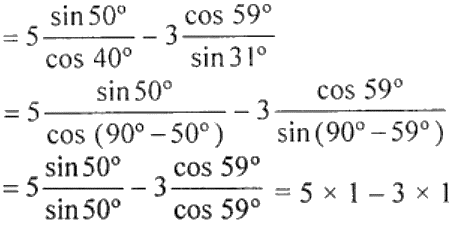= 5 – 3

= 1

9. Prove that: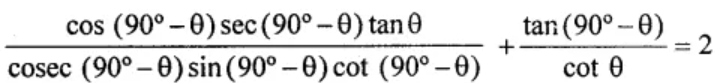Solution: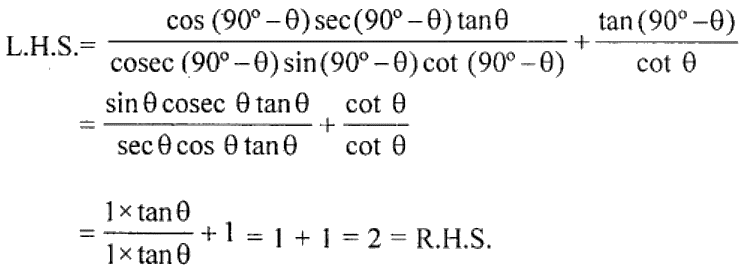Thus, L.H.S. = R.H.S.

Hence proved.

10. When 0° < A < 90°, solve the following equations:
(i) sin 3A = cos 2A
(ii) tan 5A = cot A

Solution:

(i) sin 3A = cos 2A

⇒ sin 3A = sin (90° – 2A)

So,

3A = 90° – 2A

3 A + 2A = 90°

5A = 90°

∴ A = 90°/5 = 18°

(ii) tan 5A = cot A

⇒ tan 5A = tan (90° – A)

So,

5A = 90°- A

5A + A = 90°

6A = 90°

∴ A = 90°/6 = 15°

11. Find the value of θ if
(i) sin (θ + 36°) = cos θ, where θ and θ + 36° are acute angles.
(ii) sec 4θ = cosec (θ – 20°), where 4θ and θ – 20° are acute angles.

Solution:

(i) Given, θ and (θ + 36°) are acute angles

And,

sin (θ + 36°) = cos θ = sin (90° – θ) [As, sin (90° – θ) = cos θ]
On comparing, we get

θ + 36° = 90° – θ

θ + θ = 90° – 36°

2θ = 54°

θ = 54°/2

∴ θ = 27°

(ii) Given, θ and (θ – 20°) are acute angles

And,

sec 4θ = cosec (θ – 20°)

cosec (90° – 4θ) = cosec (θ – 20°) [Since, cosec (90° – θ) = sec θ]
On comparing, we get

90° – 4θ = θ – 20°

90° + 20° = θ + 4θ

5θ = 110°

θ = 110°/5

∴ θ = 22°

12. In the adjoining figure, ABC is right-angled triangle at B and ABD is right angled triangle at A. If BD ⊥ AC and BC = 2cm, find the length of AD.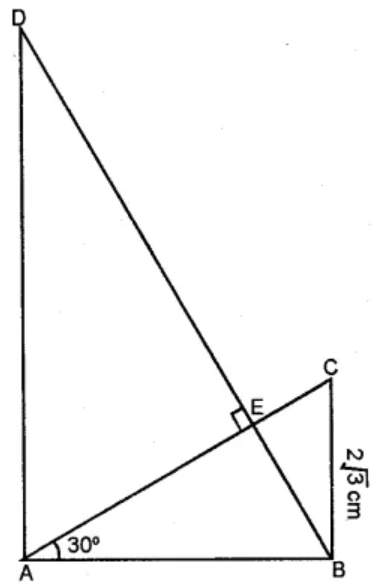Solution:

Given, ∆ABC and ∆ABD are right angled triangles in which ∠A = 90° and ∠B = 90°

And,

BC = 2√3 cm. AC and BD intersect each other at E at right angle and ∠CAB = 30°.

Now in right ∆ABC, we have

tan θ = BC/AB

⇒ tan 30° = 2√3/ AB

⇒ 1/√3 = 2√3/ AB

⇒ AB = 2√3 × √3 = 2 × 3 = 6 cm.

In ∆ABE, ∠EAB = 30° and ∠EAB = 90°

Hence,

∠ABE or ∠ABD = 180° – 90° – 30°

= 60°

Now in right ∆ABD, we have